### Coupled oscillations, pendula

#### Problem:

A pendulum is composed of two masses, 3m and m, and two strings of equal length L as shown.At t = 0 the system is released from rest with the upper (heavier) mass not displaced from its equilibrium position and the lower mass displaced to the right a distance a.
x2(0) = 0, x1(0) = a << L.
Find an expression for the subsequent motion of the lower mass, x1(t).

Solution

• Concepts:
Small, coupled oscillations; normal modes
L = ½∑ij[Tij(dqi/dt)(dqj/dt) - kijqiqj]  with Tij = Tji,  kij = kji
Solutions of the form qj = Re(Ajeiωt) can be found.  We can find the ω2 from det(kij - ω2Tij) = 0.
• Reasoning:
We are asked to find the motion of the masses for small displacements.
• Details of the calculations:
Let Φ1 and Φ2 be the generalized coordinates.  Then
x2 = LsinΦ2, x1 = L(sinΦ1 + sinΦ2),
y2 = LcosΦ1, y1 = L(cosΦ1 + cosΦ2).
For small displacements we have sinΦ = Φ, cosΦ = 1 - Φ2/2.
The kinetic energy of the system is
T = (3/2)m[(dx2/dt)2 + (dy2/dt)2] + ½m[(dx1/dt)2 + (dy1/dt)2]
Let us only keep terms up to second order in Φ and dΦ/dt.  Then
(dx2/dt)2 + (dy2/dt)2 = L2(dΦ2/dt)2,
(dx1/dt)2 + (dy1/dt)2 = L2[(dΦ2/dt)2 + (dΦ1/dt)2 + 2(dΦ2/dt)(dΦ2/dt)].
T = (3/2)mL2(dΦ2/dt)2 + ½mL2[(dΦ2/dt)2 +(dΦ1/dt)2 + 2(dΦ1/dt)(dΦ2/dt)].
The potential energy of the system is
U = -mgy1 - 3mgy2  = (mgL/2)(Φ12 + 4Φ22) + constant.
We have:
T = ½∑ij Tij(dΦi/dt)(dΦj/dt),   U = ½∑ij kijΦiΦj.
T11 = mL2,  T22 = 4mL2, T12 = T21 = mL2,
k11 = mgL, k22 = 4mgL, kij(i ≠ j) = 0.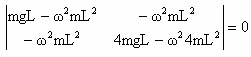.

ω2 = (g/L)(8 ± 4)/6, ωa2 = 2(g/L), ωb2 = (2/3)(g/L).
Φi = Aiexp(iωαt)
For mode a:  A2 = -A1
For mode b:  A2 = A1
Most general motion for a system starting from rest:
Φ1 = Aexp(iωat) + Bexp(iωbt),  Φ2 = -(A/2)exp(iωat) + (B/2)exp(iωbt).
x1 = L(A/2)exp(iωat) + L(3B/2)exp(iωbt),  x2 = -L(A/2)exp(iωat) + L(B/2)exp(iωbt),
x1 = A'exp(iωat) + 3B'exp(iωbt),  x2 = -A'exp(iωat) + B'exp(iωbt).
Initial conditions:  A' + 3 B' = a,  -A' + B' = 0  -->  A' = B' = a/4.
Subsequent motion of the lower mass: x1(t) = (a/4)cos ωat + (3a/4)cos ωbt.

#### Problem:

A uniform thin rod of length 3l/2 and mass m is supported at one end A by a weightless string of length l under the gravitational force near the earth surface.
(a)  Calculate the normal modes and normal frequencies of small oscillations for motion in a vertical plane.
(b)  Now the end point A is slowly displaces by a small amount δ, (without gaining any kinetic energy.)  The system is then released from rest and allowed to move freely.  What is the subsequent motion?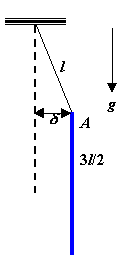Solution:

• Concepts:
Small oscillations:
L = ½∑ij[Tij(dqi/dt)(dqj/dt) - kijqiqj]  with Tij = Tji,  kij = kji
Solutions of the form qj = Re(Ajeiωt) can be found.
For a particular frequency ωα we solve
j[kij - ωα2Tij]A = 0
to find the A.  The most generαl solution for eαch coordinαte qj is α sum of simple hαrmonic oscillαtions in αll of the frequencies ωα.
qj = Re∑α(CαAjαexp(iωαt)).
• Reasoning:
We are asked to find the normal modes for small displacements.
• Details of the calculations: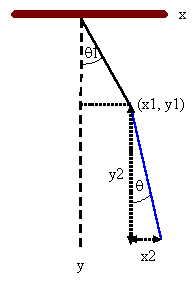(a)  The position of the CM of the rod is X = x1 + x2/2, Y = y1 + y2/2.
For the rod we have:
T = ½m[(dx1/dt + ½ dx2/dt)2 + (dy1/dt + ½ dy2/dt)2]  + ½I(dθ/dt)2.
T is the kinetic energy of the motion of the CM and the motion about the CM.
U = -mg(y1 + y2/2) + constant.
For small oscillations x1 = lsinθ1 ~lθ1, x2 = (3/2)lsinθ ~ (3/2)lθ.
y1 = lcosθ1 ~ l(1 - θ12/2), y2 = (3/2)lcosθ ~ (3/2)l(1 - θ2/2).
To second order in the small quantities we have:
T = ½ml2((dθ1/dt)22(dθ/dt)2+(3/2)(dθ1/dt)(dθ/dt)+½(3/16)ml2(dθ/dt)2)
= ½ml2((dθ1/dt)2+¾(dθ/dt)2+(3/2)(dθ1/dt)(dθ/dt))
= ½∑Tijqiqj.
q1 = θ1, q2 = θ, T11 = ml2, T22 = ¾ml2, T12 = T21 = ¾ml2.
U = mgl[(q12/2) +¾l(q22/2)] = ½∑kijqiqj.
k11 = mgl, k22 = ¾mgl, k12 = k21 = 0.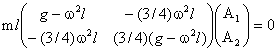.

We have a solution if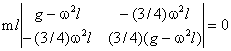.

¾(g-ω2l)2 -(9/16)ω4l2 = 0,  ω4 - 8(g/l)ω2 + 4g2/l = 0.
ω2 = [4 ± (12)½]g/l.
mode 1:  ω12 = [4 + (12)½]g/l.
(g - [4 + (12)½]g)A1 - ¾[4 + (12)½]gA2 = 0.  A1 = -0.866 A2.
mode 2:  ω22 = [4 - (12)½]g/l.
(g - [4 - (12)½]g)A1 - ¾[4 - (12)½]gA2 = 0.  A1 = 0.866 A2.

(b)  The most general solution is qj = Re∑α(CαAexp(iωαt)).
θ1 = Re(c1exp(iω1t) + c2exp(iω2t)),  θ = (1/0.866)Re(-c1exp(iω1t) + c2exp(iω2t)).
Initial conditions:
θ1(0) = δ/l,  θ(0) = 0,  dθ1/dt = dθ/dt = 0 at t = 0.
Re(c1 + c2) = δ/l,  Re(c1 - c2) = 0,  Re(c1) = Re(c2) = δ/(2l).
Re(iω1c1 + iωc2) = 0,  Re(-iω1c1 + iωc2) = 0.
Im(ω1c1 + ωc2) = 0,  Im(ω1c1 - ωc2) = 0,  Im(c1) = Im(c2) = 0.
θ1(t) = (δ/(2l))[cosω1t + cosω2t],  θ(t) = (δ/(2*0.866*l))[cosω1t - cosω2t].